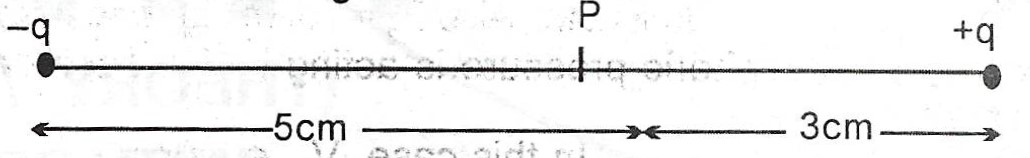# (a) Explain what is meant by: (i) electric field intensity ; (ii) electric lines of force. (b) Two similar but opposite point charges -q and +q each of magn...

Question 1

(a) Explain what is meant by: (i) electric field intensity ; (ii) electric lines of force.

(b) Two similar but opposite point charges -q and +q each of magnitude $$5 \times 10^{-8} C$$ are seperated by a distance of 8.0cm in vacuum as shown in the diagram below.Calculate the magnitude and direction of the resultant electric field intensity E at the point P. Draw the lines of force due to this system of charges. [Take $$\frac{1}{4 \pi \varepsilon _{0}}$$]

(c)